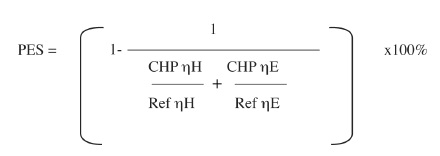# Electricity Regulation Act 1999

F453[SCHEDULE 3

Combined Heat and Power

1. Subject to paragraph 2, electricity production from combined heat and power shall be calculated as follows:

(a) in the case of combined cycle gas turbine with heat recovery or in the case of steam condensing extraction turbine with an annual overall efficiency of 80 per cent or greater, the total annual electricity production of the unit measured at the outlet of the main generators shall be considered to be electricity produced from combined heat and power;

(b) in the case of any other technology or technologies which satisfy the definition of combined heat and power and which have an annual overall efficiency of 75 per cent or greater, the total annual electricity production of the unit measured at the outlet of the main generators shall be considered to be electricity produced from combined heat and power; and

(c) in the case of combined heat and power units with an annual overall efficiency below the values referred to in subparagraphs (a) and (b), the electricity produced from combined heat and power is calculated as follows:

E CHP = H CHP . C

where:

 E CHP is electricity production from combined heat and power. C is the actual power to heat ratio of the unit. H CHP is the amount of useful heat from combined heat and power (calculated as total heat production minus any heat produced in separate boilers or by live steam extraction from the steam generator before the turbine).

2. If a share of the energy content of the fuel input to the combined heat and power process is recovered in chemicals and recycled this share can be subtracted from the fuel input before calculating the overall efficiency used in paragraph 1.

3. The relative amount of primary energy savings provided by combined heat and power production shall be calculated as follows:Where:

 PES is primary energy savings. CHPηH is the heat efficiency of the combined heat and power, defined as annual useful heat output divided by the fuel input used to produce the sum of useful heat output and electricity production from combined heat and power. RefηH is the efficiency reference value for separate heat production determined in accordance with section 7(6). CHPηE is the electrical efficiency of the combined heat and power, defined as annual electricity production from combined heat and power divided by the fuel input used to produce the sum of useful heat output and electricity production from combined heat and power. Where a combined heat and power unit generates mechanical energy, the annual electricity from combined heat and power may be increased by the amount of electricity which is equivalent to that of mechanical energy. RefηE is the efficiency reference value for separate electricity production determined in accordance with section 7(6).

4. Values used for calculation of electricity from combined heat and power and the amount of primary energy savings provided by combined heat and power shall be determined on the basis of

(a) the expected or actual operation of the unit under normal conditions of use, or

(b) in the case of micro-combined heat and power units, certified values.

5. The principles in accordance with which efficiency reference values for separate electricity and heat production may be determined under an order made under section 7(6) are as follows:

(a) the comparison with separate electricity production shall be based on the principle that the same fuel categories are compared and the comparison is with the best available and economically justifiable technology for separate production of heat and electricity on the market in the year of construction of the power unit concerned;

(b) the efficiency reference values for units older than 10 years of age shall be fixed on the reference values of units of 10 years of age;

(c) the efficiency reference values for separate electricity production and heat production shall, if necessary, be calibrated to reflect Irish climatic conditions.]

Annotations

Amendments:

F453

Inserted (29.07.2009) by Energy (Miscellaneous Provisions) Act 2006 (40/2006), s. 6(d) and sch., S.I. No. 298 of 2009.